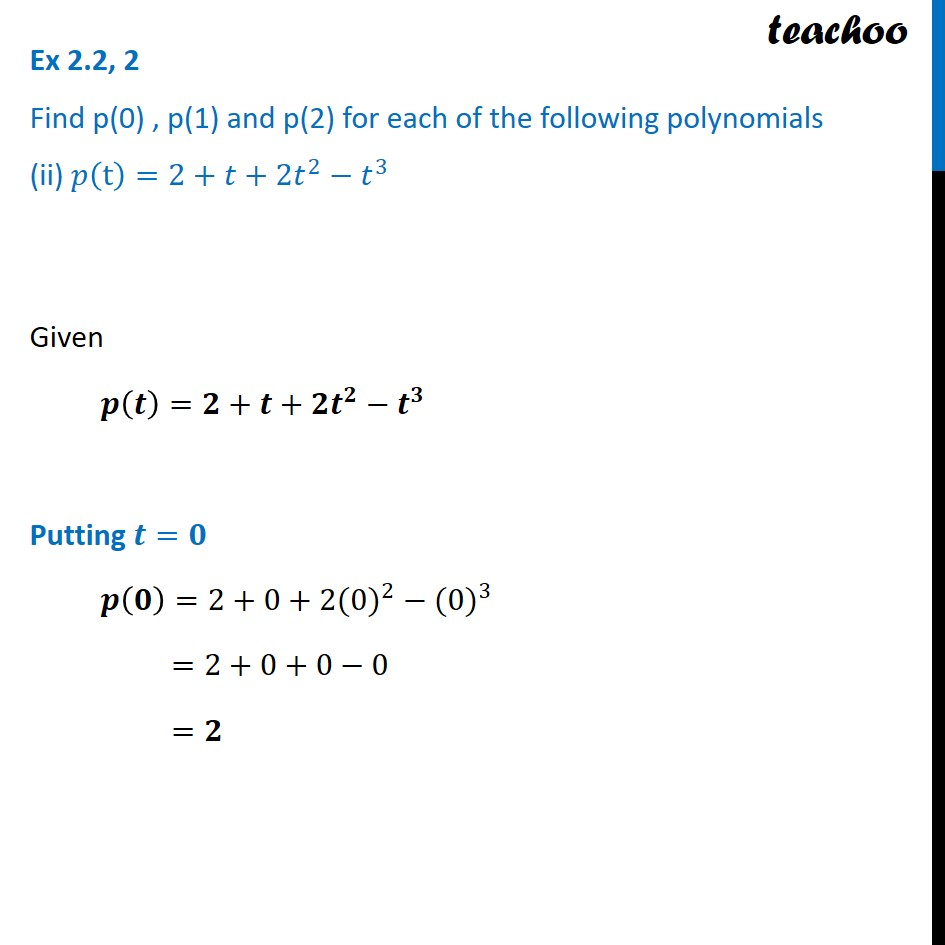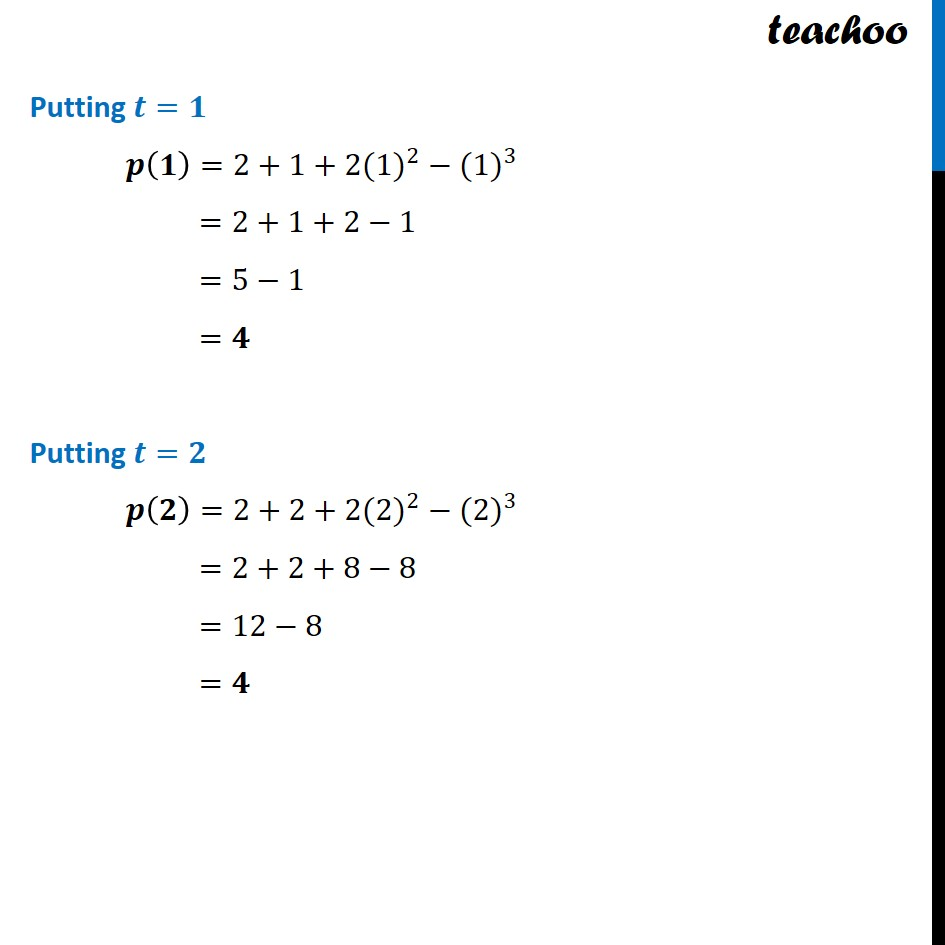Ex 2.2

Chapter 2 Class 9 Polynomials
Serial order wiseLearn in your speed, with individual attention - Teachoo Maths 1-on-1 Class

### Transcript

Ex 2.2, 2 Find p(0) , p(1) and p(2) for each of the following polynomials (ii) 𝑝(t)=2+𝑡+2𝑡^2−𝑡^3 Given 𝒑(𝒕)=𝟐+𝒕+𝟐𝒕^𝟐−𝒕^𝟑 Putting 𝒕=𝟎 𝒑(𝟎)=2+0+2(〖0)〗^2−〖(0)〗^3 =2+0+0−0 =𝟐 Putting 𝒕=𝟏 𝒑(𝟏)=2+1+2(〖1)〗^2−〖(1)〗^3 =2+1+2−1 =5−1 =𝟒 Putting 𝒕=𝟐 𝒑(𝟐)=2+2+2(〖2)〗^2−〖(2)〗^3 =2+2+8−8 =12−8 =𝟒